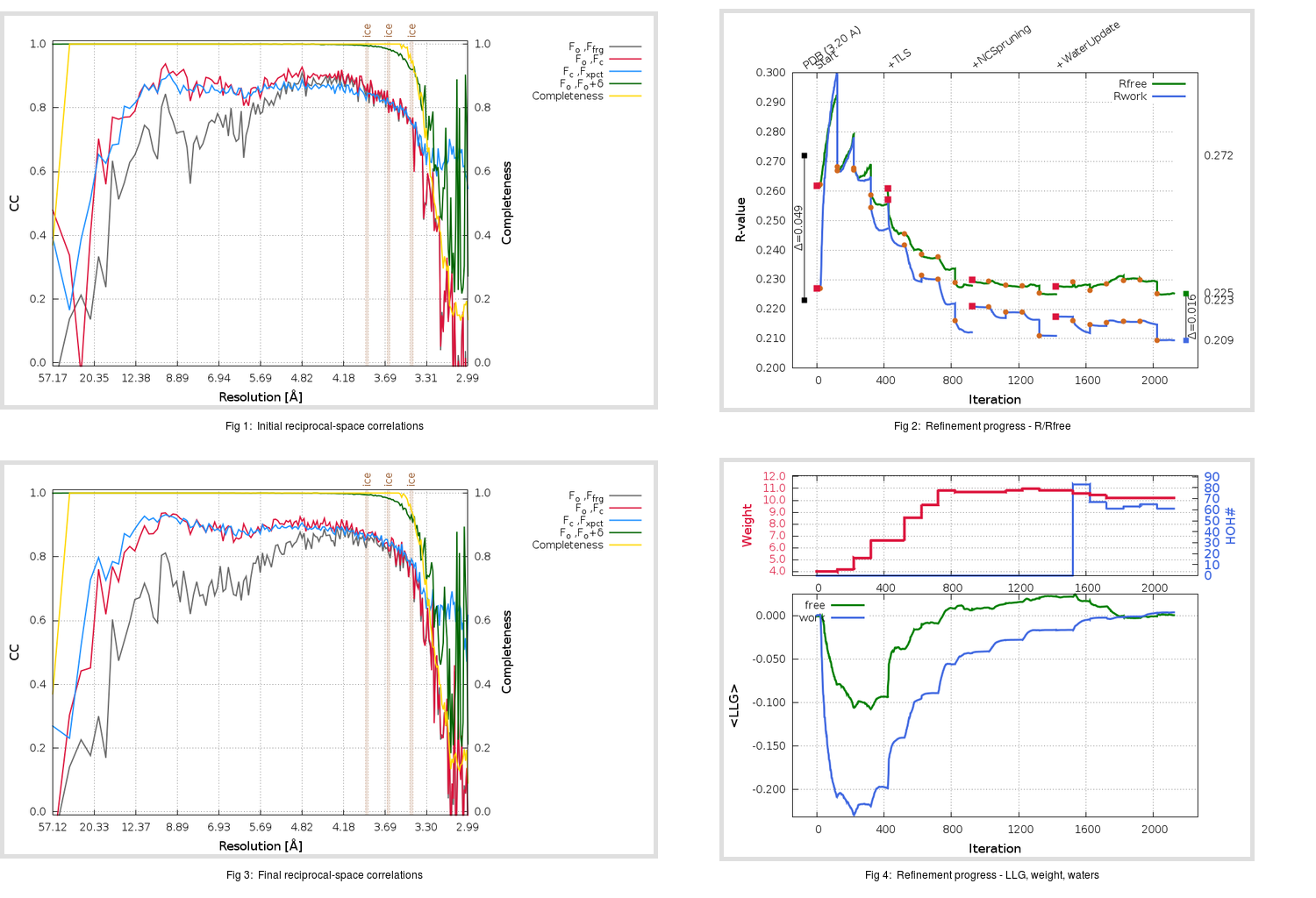Content:

## Deposited

` `
 Date deposited Date data collection Resolution R, Rfree 20090902 20090321 3.20 0.2200 0.2720

Molprobity (CCP4 7.0 version) summary:

```Ramachandran outliers =   9.02 %
favored =  77.05 %
Rotamer outliers      =  22.94 %
C-beta deviations     =     9
Clashscore            =  48.17
RMS(bonds)            =   0.0102
RMS(angles)           =   1.53
MolProbity score      =   3.97
Resolution            =   3.20
R-work                =   0.2200
R-free                =   0.2720
```

```Number of waters      =     0

<B> (all atoms) =   86.62 ( sd =   19.08 ) for       9512 non-hydrogen atoms
<B>   (protein) =   86.28 ( sd =   18.85 ) for       9396 non-hydrogen atoms
<B>     (water) =    0.00 ( sd =    0.00 ) for          0 non-hydrogen atoms
<B>    (others) =  113.77 ( sd =   18.47 ) for        116 non-hydrogen atoms

B min/max       (all non-hydrogen atoms) =   50.64 /  161.56
B min/max   (protein non-hydrogen atoms) =   50.64 /  161.56
B min/max     (water non-hydrogen atoms) =    0.00 /    0.00
B min/max     (other non-hydrogen atoms) =   77.02 /  152.60
```

## BUSTER (re-)refinement

` `

Molprobity (CCP4 7.0 version) summary:

```Ramachandran outliers =   1.67 %
favored =  93.57 %
Rotamer outliers      =  11.04 %
C-beta deviations     =     5
Clashscore            =   6.69
RMS(bonds)            =   0.0126
RMS(angles)           =   1.63
MolProbity score      =   2.58
Resolution            =   2.99
R-work                =   0.2095
R-free                =   0.2253
```

```Number of waters      =    61

<B> (all atoms) =  108.59 ( sd =   23.68 ) for       9573 non-hydrogen atoms
<B>   (protein) =  108.56 ( sd =   23.55 ) for       9396 non-hydrogen atoms
<B>     (water) =   88.07 ( sd =   31.70 ) for         61 non-hydrogen atoms
<B>    (others) =  121.89 ( sd =   20.62 ) for        116 non-hydrogen atoms

B min/max       (all non-hydrogen atoms) =   57.09 /  300.00
B min/max   (protein non-hydrogen atoms) =   73.92 /  232.71
B min/max     (water non-hydrogen atoms) =   57.09 /  300.00
B min/max     (other non-hydrogen atoms) =   93.87 /  157.72
```

Refinement progression:Results:

` `
 File Remark 3IWM_aB_refine.01_04_refine.pdb.gz exact refinement commands are in header 3IWM_aB_refine.01_04_refine.mtz.gz including original deposited data and several re-refinement map coefficients 3IWM_aB_refine.01_04_BUSTER_model.cif.gz including any non-standard compound restraints 3IWM_aB_refine.01_04_BUSTER_refln.cif.gz